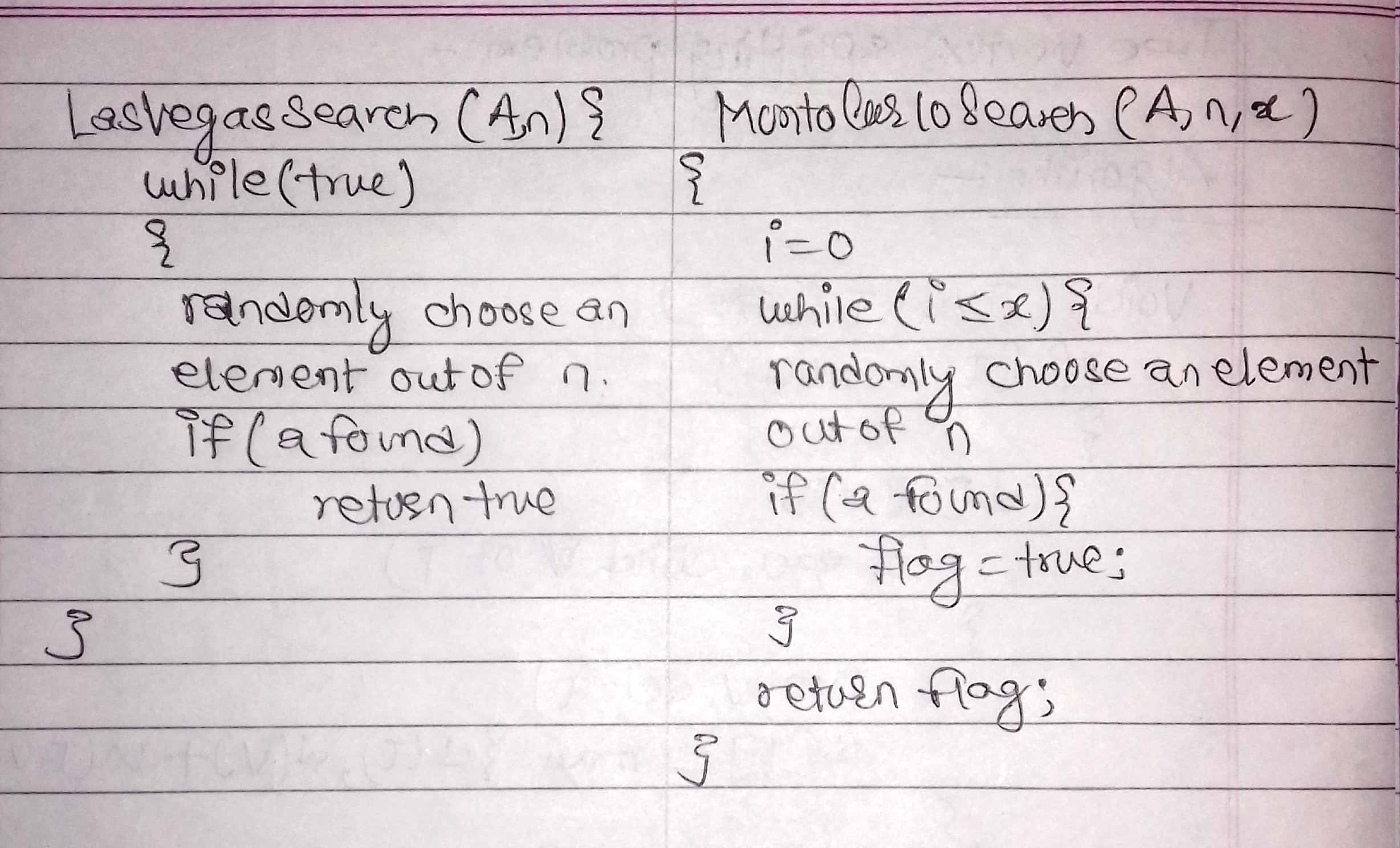December 10, 2018 Root User

### Randomized Algorithm:

• A probabilistic algorithm is an algorithm where the result and/or the way the result is obtained depend on chance. These algorithms are also sometimes called randomized algorithms.

• It makes use of randomizer(random number generator) to make some decision in a algorithm.

• The output and/or execution time of a randomized algorithm could also differ from run to run for the same input.

• It can be categorized into:

1. Las vegas algorithm

2. Monte carlo algorithm

Las vegas Algorithm

• this algorithm always same (correct) output for the same input.

• Execution time of this algorithm is characterized as a random variable.

• The execution time depends on the output of randomizer.

Monte Carlo Algorithm

• Monte carlo is the algorithm whose output might differ from run to run for the same input.

• It may generate the wrong answer sometimes that depends on the output of the randomizer.

• The execution time is fixed.Prosperous Nepal is possible only from technological innovation.